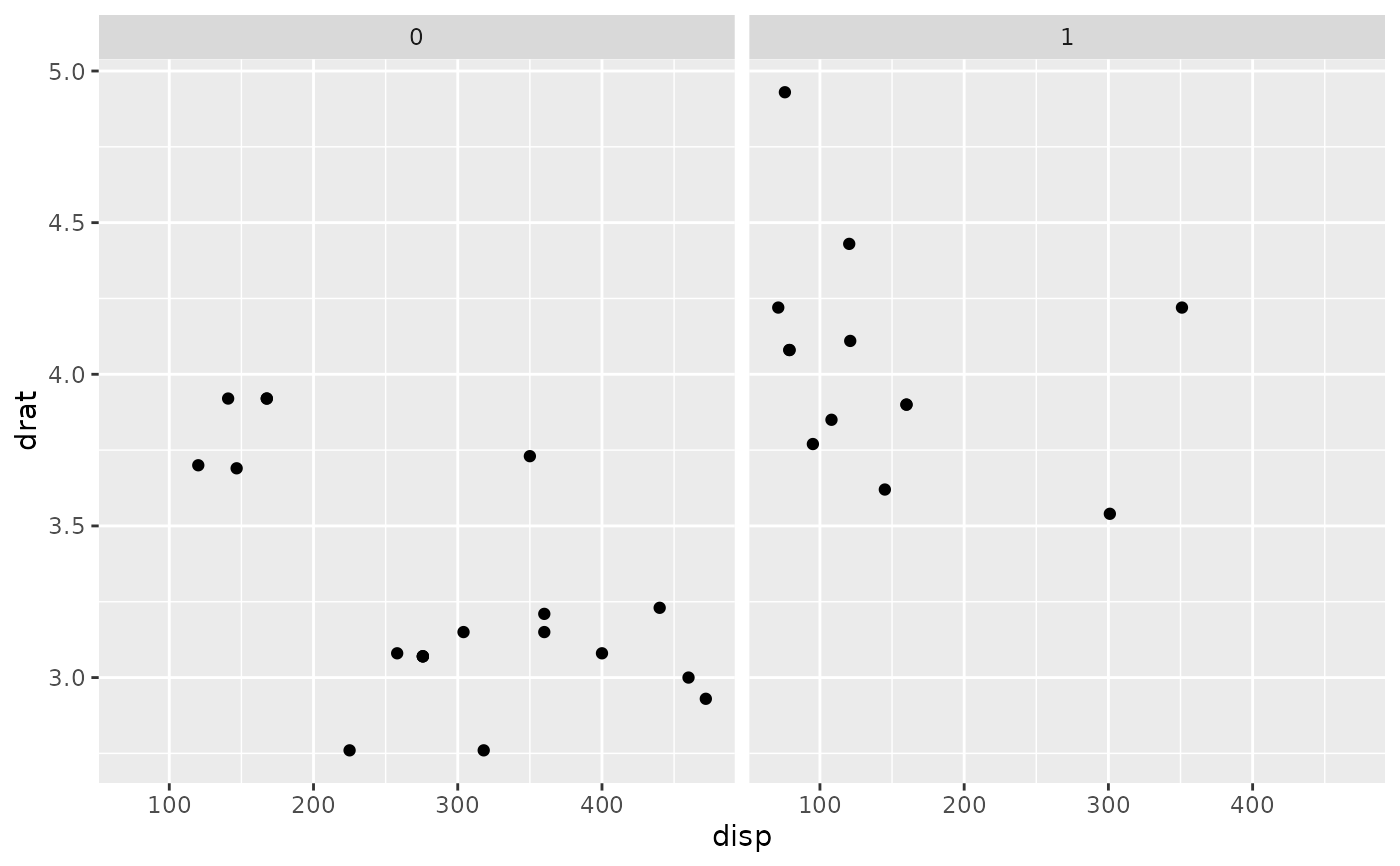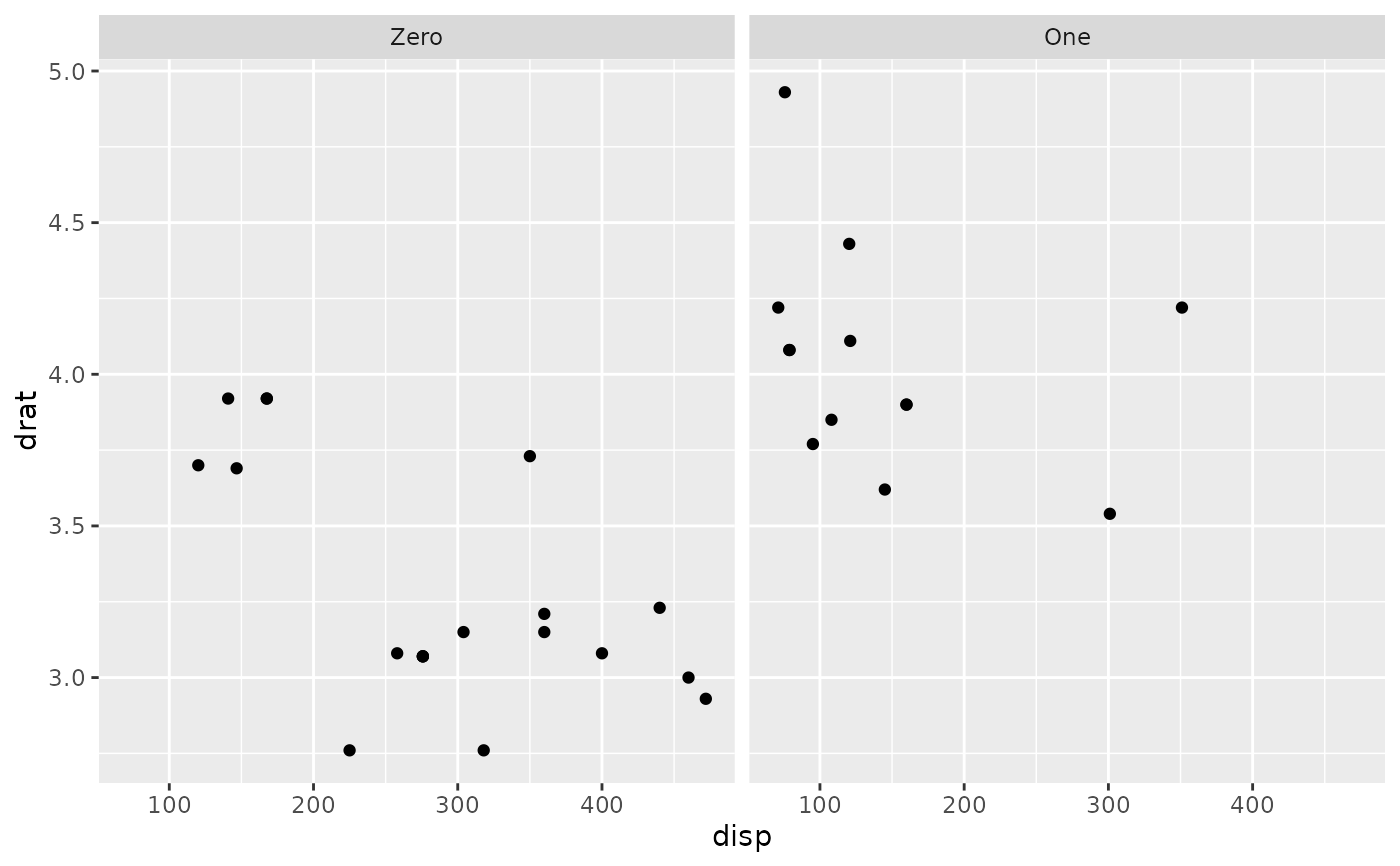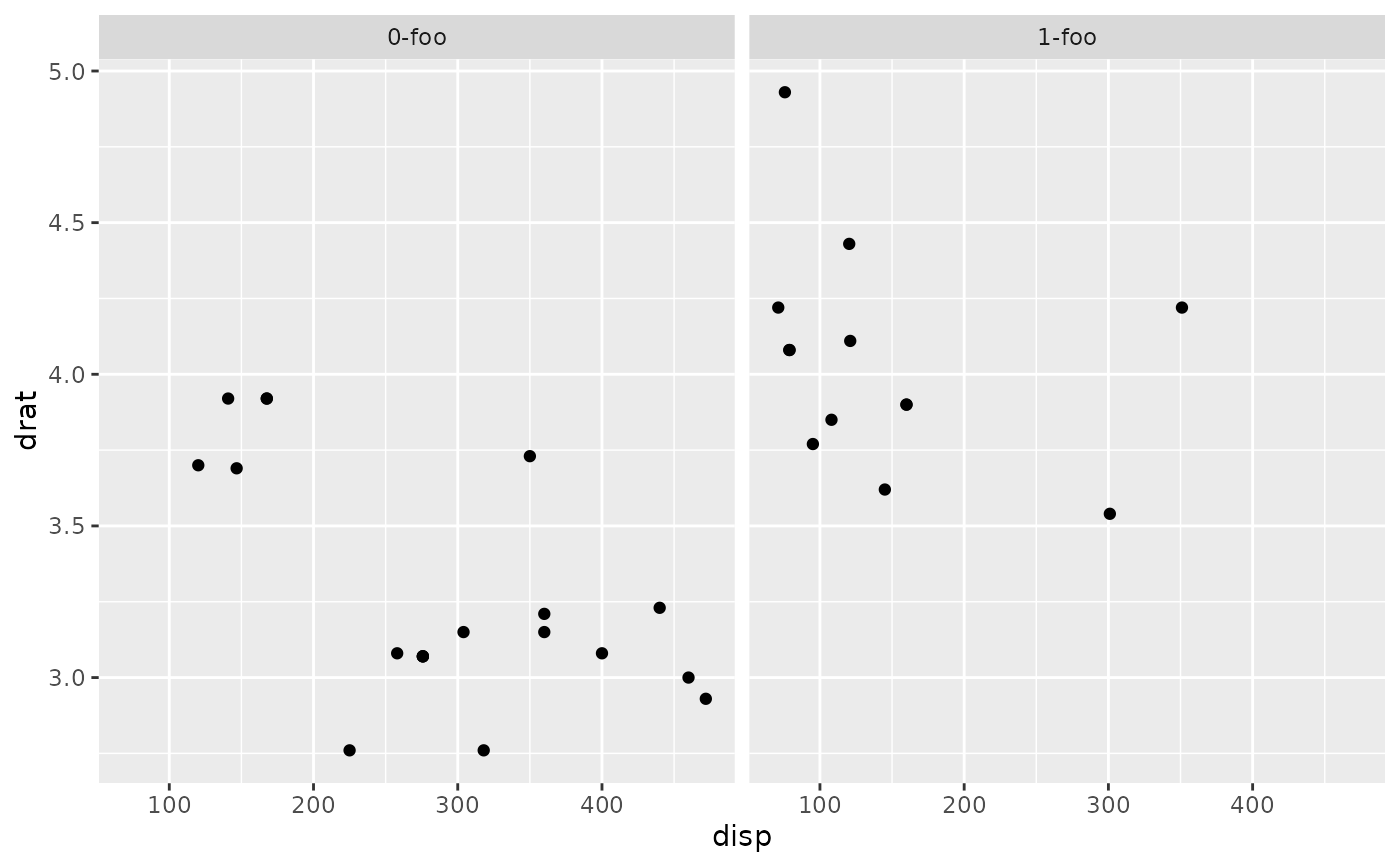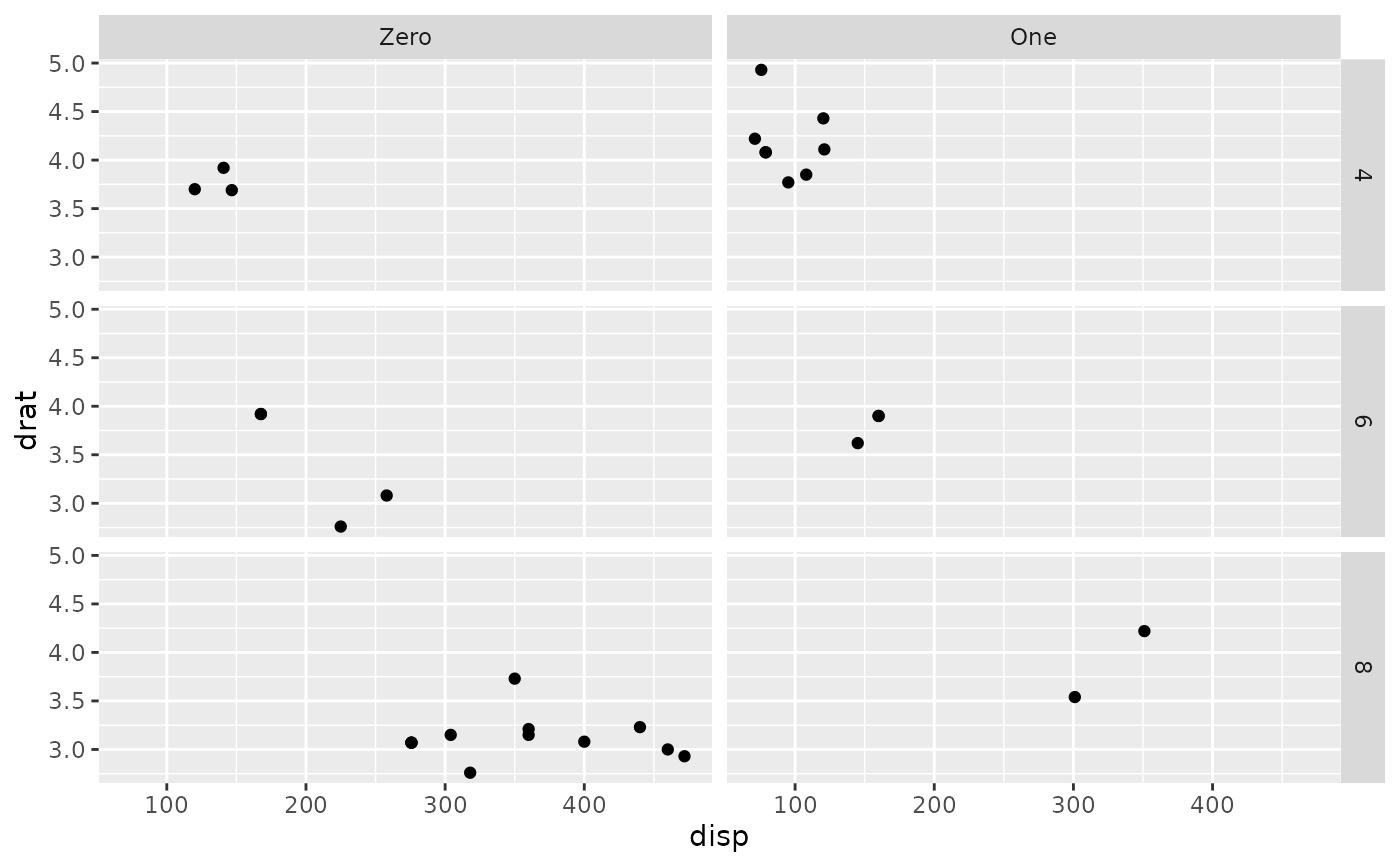This transforms objects to labeller functions. Used internally by labeller().

## Usage

as_labeller(x, default = label_value, multi_line = TRUE)

## Arguments

x

Object to coerce to a labeller function. If a named character vector, it is used as a lookup table before being passed on to default. If a non-labeller function, it is assumed it takes and returns character vectors and is applied to the labels. If a labeller, it is simply applied to the labels.

default

Default labeller to process the labels produced by lookup tables or modified by non-labeller functions.

multi_line

Whether to display the labels of multiple factors on separate lines. This is passed to the labeller function.

labeller(), labellers

## Examples

p <- ggplot(mtcars, aes(disp, drat)) + geom_point()
p + facet_wrap(~am)# Rename labels on the fly with a lookup character vector
to_string <- as_labeller(c(0 = "Zero", 1 = "One"))
p + facet_wrap(~am, labeller = to_string)# Quickly transform a function operating on character vectors to a
# labeller function:
appender <- function(string, suffix = "-foo") paste0(string, suffix)
p + facet_wrap(~am, labeller = as_labeller(appender))# If you have more than one faceting variable, be sure to dispatch
# your labeller to the right variable with labeller()
p + facet_grid(cyl ~ am, labeller = labeller(am = to_string))